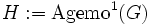# Characteristic-isomorph-free not implies normal-isomorph-free in finite

This article gives the statement and possibly, proof, of a non-implication relation between two subgroup properties. That is, it states that every subgroup satisfying the first subgroup property (i.e., characteristic-isomorph-free subgroup) need not satisfy the second subgroup property (i.e., normal-isomorph-free subgroup)
View a complete list of subgroup property non-implications | View a complete list of subgroup property implications
EXPLORE EXAMPLES YOURSELF: View examples of subgroups satisfying property characteristic-isomorph-free subgroup but not normal-isomorph-free subgroup|View examples of subgroups satisfying property characteristic-isomorph-free subgroup and normal-isomorph-free subgroup

## Statement

We may have a group with a characteristic subgroup such that there are no other characteristic subgroups isomorphic to it, but there are other normal subgroups isomorphic to it.

## Proof

### Example of an Abelian group

Let$p$ be any prime, and let$G := \mathbb{Z}/p\mathbb{Z} \times \mathbb{Z}/p^2\mathbb{Z}$. In other words,$G$ is a direct product of cyclic groups of order$p$ and$p^2$ respectively.

Consider the subgroup$H := \operatorname{Agemo}^1(G)$: the set of all elements of$G$ that can be written as$p^{th}$ powers. This set is$0 \times p\mathbb{Z}/p^2\mathbb{Z}$.

•$H$ is a characteristic subgroup: It is defined as the set of$p^{th}$ powers, so it is clearly invariant under conjugation.
• There is no other characteristic subgroup of$G$ of order$p$: All the subgroups of order$p$ in$G$ lie inside$\Omega_1(G) = \mathbb{Z}/p\mathbb{Z} \times p\mathbb{Z}/p^2\mathbb{Z}$. Further, automorphisms of the form$(a,b) \mapsto (a + b,b)$ permute all the other subgroups.
• There are other normal subgroups of$G$ of order$p$: In fact, there are$p$ of them: the other$p$ subgroups of$\Omega_1(G)$.

Thus,$H$ is characteristic-isomorph-free in$G$ but is not normal-isomorph-free in$G$.